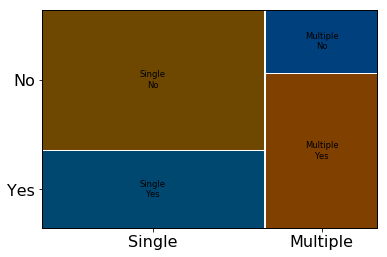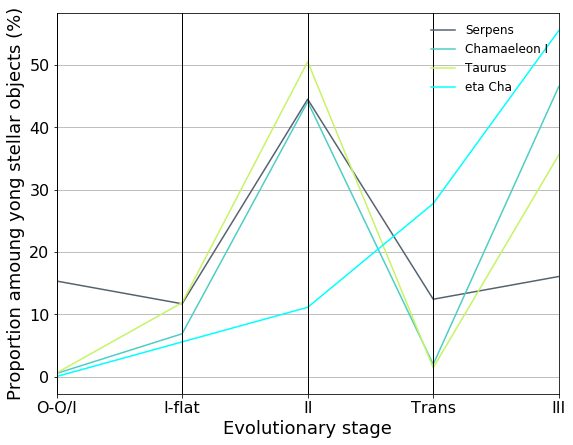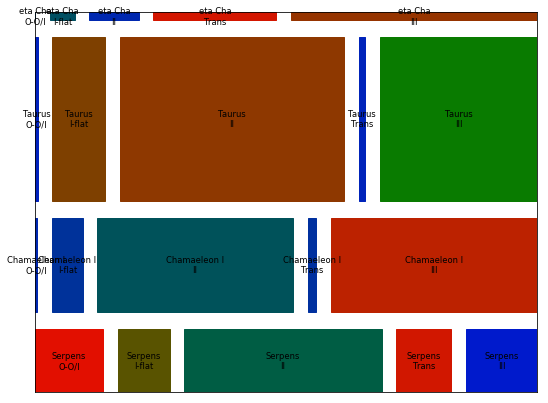### Star Formation¶

Author Wing-Fai Thi

Version 1.1 19/3/2018 add Bonamente's book in the reference

1.2 1/4/2018 add mosaic and parallel coordinate plots and references

This notebook concerns two of the exercises in the book Modern Statistical Methods for Astronomy by E. Feigelson and J Babu. It shows that Data Science is not always about large amount of data. The results are howver very insightfull in the field of star- and protoplanetary- formation and early evolution.

#### Aim of the notebook¶

• Explore statistical methods applied to star-formation in nearby molceular clouds: contingency tables, hypothesis testings. More precisely, we will
• compare the χ2 test and Fisher exact test to evaluate whether multiple protostars are more likely to produce jets than single protostars.
• use the protostellar jet data to investigate various approaches to the binomial proportion problem
• analyse a contingency table of young star class membership in four nearby star-forming regions and derive an evolutionary scenario.

#### Reference¶

• Massimiliano Bonamente, Statistics and Analysis of Scientific Data, 2nd edition, Springer
• David Meyer, Achim Zeilies, Jurt Hornik Vizualising Contingency Tables in C. Chen, W. Haerdle, A. Unwin editors, Handbook of data Visualization, Springer
• Alfred Inselberg Parallel Coordinates: Visualization, Exploration and Classification of high-Dimensional Data in Handbook of data Visualization, Springer
In :
import numpy as np
import pandas as pd
import matplotlib as mpl
import matplotlib.pyplot as plt
from matplotlib import rcParams
from statsmodels.graphics.mosaicplot import mosaic
from pandas.plotting import parallel_coordinates
# get configuration file location
#print (mpl.matplotlib_fname())

# get configuration current settings
#print (mpl.rcParams)

# Change the default settings
mpl.rcParams.update({'figure.figsize': (9.0, 7.0),
'figure.dpi' : 300, # 300 dpi for print
'font.size': 14,
'legend.frameon': False,
'legend.fontsize' : 12,
'xtick.labelsize' : 16,
'ytick.labelsize' : 16,
'axes.labelsize'  : 18
})


One can create a mosaic plot from a contingency table. A mosiac plot allows to visualize multivariate categorical data in a rigorous and informative way.

In :
# Load the 2x2 contingency table


#### Part 1¶

The first part deals with the question: Does jets related to the multiplicity of the central object?

The table below contains in a contingency/frequency table format the result of a survey of jets.

In :
df

Out:
Multiplicity No Yes Total
0 Single 9 5 14
1 Multiple 2 5 7
2 Total 11 10 21
In :
# multiplicity statistics
print "General jet frequency:",10./21.

General jet frequency: 0.47619047619

In :
print "Single protostar jet frequency:",5./14.

Single protostar jet frequency: 0.357142857143

In :
print "Multiple protostar jet frequency:",5./7.

Multiple protostar jet frequency: 0.714285714286


By looking at the simple frequencies, one is tempted to conclude that multiple protostars tend to show jets more often than single protostars. Is that conclusion correct? We will use the analysis of the contingency/frequency table to help us.

In :
from scipy.stats import fisher_exact, chi2_contingency
# we extract the table as numpy array
contingency_table = df[['No','Yes']]
contingency_table = np.array(contingency_table.drop())

In :
# hypothesis test of independence of the observed frequencies
# in the contingency table
# Expected = row total x column total / all cell total
chi2,p_chi2,dof,expected = chi2_contingency(contingency_table)


We perform a Chi-square test.

In :
print "Chi-square p-value is",p_chi2

Chi-square p-value is 0.27954138007236257

In :
dfm = df.set_index('Multiplicity')
dfm = dfm[['Yes','No']]
dfm.drop('Total',inplace=True)
dfm.columns.names =['Jet?']
dfm

Out:
Jet? Yes No
Multiplicity
Single 5 9
Multiple 5 2
In :
mosaic(dfm.stack(),gap=0.01,statistic=True)
plt.show()In :
df_expected=pd.DataFrame(expected,columns=['No','Yes'])
df_expected=pd.concat((df['Multiplicity'].drop(2),df_expected),axis=1)
df_expected

Out:
Multiplicity No Yes
0 Single 7.333333 6.666667
1 Multiple 3.666667 3.333333
In :
oddsratio , p_fisher = fisher_exact(contingency_table)

In :
# Fisher exact test is appropriate for small-n samples.
print "Fisher's test p-value is", p_fisher

Fisher's test p-value is 0.18266253869969057


The probability that we would observe this or an even more imbalanced ratio by chance is about 18.2 % (27.9% for the chi-square test). A commonly used significance level is 5%. if we adopt that, we can therefore conclude that our observed imbalance is not statistically significant: no significant effect of protostellar multiplicity on jet formation is present. The conlusion holds for the particular values in the table. The analyiss does not prevent bias in the sampling.

The standardized residual table is more meaningful and is quite simple to interpret. The standardized residuals are simply the deviation from the expected value in standard deviation. A strandar residual is the residual value divided by the square-root of the expected value.

In :
# standardized residuals see statisticshowto.com
res = contingency_table-expected
st_res = res/np.sqrt(expected)
df_res=pd.DataFrame(res,columns=['No','Yes'])
df_res=pd.concat((df['Multiplicity'].drop(2),df_res),axis=1)
print "Resisuals"
df_res

Resisuals

Out:
Multiplicity No Yes
0 Single 1.666667 -1.666667
1 Multiple -1.666667 1.666667

All the standardized residual values are within 2-sigma deviation (in both directions). The higher occurence of jet for multiple protostars is most likely be due to pure chance.

In :
# Wilson's estimator
count = 5.
total = 14.
nbar = total + 4.
pw = (count+2.)/nbar

In :
from scipy.stats import norm
confidence = 0.95
alpha = 1.-confidence
z=norm.ppf(1-0.5*alpha)


We use the Wilson's estimator (Estimation of the ratio of binomial random variables, see Feigelson & Babu, p.80)

In :
print "z-score=",z
interval=z*np.sqrt(pw*(1-pw)/nbar)
print pw,"+/-",interval

z-score= 1.959963984540054
0.388888888889 +/- 0.22520845746331428


The Wilson estimator confirms the previous conclusion.

#### Part 2¶

Aim: Test the null hypotheses that that the distribution of evolutionary classes is the same in all star-forming regions.

In :
df2=pd.read_csv("ProtostatPopulations.csv")
df2

Out:
Cloud O-O/I I-flat II Trans III Reference
0 Serpens 21 16 61 17 22 Winston et al. 2007
1 Chamaeleon I 1 14 90 4 95 Luhman et al. 2008
2 Taurus 2 42 179 5 126 Luhman et al. 2010
3 eta Cha 0 1 2 5 10 Sicilia-Aguilar et al. 2009

One can easily create by hand her/his own csv file with the values in the table above.

In :
Type = ['O-O/I','I-flat','II','Trans','III']
RC1 = np.array(df2[Type])
from scipy.stats.contingency import margins
m0, m1 = margins(RC1)

In :
row_proportion=pd.DataFrame(RC1/(1.*m0)*100.,columns=Type)
row_proportion=pd.concat((df2['Cloud'],row_proportion),axis=1)
row_proportion

Out:
Cloud O-O/I I-flat II Trans III
0 Serpens 15.328467 11.678832 44.525547 12.408759 16.058394
1 Chamaeleon I 0.490196 6.862745 44.117647 1.960784 46.568627
2 Taurus 0.564972 11.864407 50.564972 1.412429 35.593220
3 eta Cha 0.000000 5.555556 11.111111 27.777778 55.555556
In :
plt.figure(figsize=(9,7))
parallel_coordinates(row_proportion,'Cloud',
color=('#556270','#4ECDC4', '#C7F464','cyan'),alpha=1.0)
plt.xlabel('Evolutionary stage')
plt.ylabel('Proportion amoung yong stellar objects (%)')
plt.show()In :
column_proportion=pd.DataFrame(RC1/(1.*m1)*100.,columns=Type)
column_proportion=pd.concat((df2['Cloud'],column_proportion),axis=1)
column_proportion

Out:
Cloud O-O/I I-flat II Trans III
0 Serpens 87.500000 21.917808 18.373494 54.838710 8.695652
1 Chamaeleon I 4.166667 19.178082 27.108434 12.903226 37.549407
2 Taurus 8.333333 57.534247 53.915663 16.129032 49.802372
3 eta Cha 0.000000 1.369863 0.602410 16.129032 3.952569
In :
chi2,p_chi2,dof,expected = chi2_contingency(RC1)


We perform a Chi-square test.

In :
print "Chi-square p-value is",p_chi2

Chi-square p-value is 8.440817353859272e-28


The p-values << 1, significant differences exist.

In :
# Expected = row total x column total / all cell total
df_expected=pd.DataFrame(expected,columns=Type)
df_expected=pd.concat((df2['Cloud'],df_expected),axis=1)
print "Expected"
df_expected

Expected

Out:
Cloud O-O/I I-flat II Trans III
0 Serpens 4.611501 14.026648 63.792426 5.956522 48.612903
1 Chamaeleon I 6.866760 20.886396 94.990182 8.869565 72.387097
2 Taurus 11.915849 36.244039 164.835905 15.391304 125.612903
3 eta Cha 0.605891 1.842917 8.381487 0.782609 6.387097

Table of expected values: Expected = row total x column total / all cell total

In :
res = RC1-expected
df_res=pd.DataFrame(res,columns=Type)
df_res=pd.concat((df2['Cloud'],df_res),axis=1)
print "Resisuals"
df_res

Resisuals

Out:
Cloud O-O/I I-flat II Trans III
0 Serpens 16.388499 1.973352 -2.792426 11.043478 -26.612903
1 Chamaeleon I -5.866760 -6.886396 -4.990182 -4.869565 22.612903
2 Taurus -9.915849 5.755961 14.164095 -10.391304 0.387097
3 eta Cha -0.605891 -0.842917 -6.381487 4.217391 3.612903

The residual table is just the contngency table values - the expected values.

In :
# standardized residuals see statisticshowto.com
st_res = res/np.sqrt(expected)

In :
df_st_res=pd.DataFrame(st_res,columns=Type)

In :
df_st_res=pd.concat((df2['Cloud'],df_st_res),axis=1)

In :
print "Standardized residuals"
df_st_res

Standardized residuals

Out:
Cloud O-O/I I-flat II Trans III
0 Serpens 7.631643 0.526899 -0.349621 4.524906 -3.816950
1 Chamaeleon I -2.238837 -1.506816 -0.512008 -1.635080 2.657821
2 Taurus -2.872549 0.956092 1.103222 -2.648697 0.034538
3 eta Cha -0.778390 -0.620915 -2.204252 4.767290 1.429567

A couple of remarks can be made from the standardized residual table. The Serpens star-forming region has 7.6 sigma more class O and O-I objects than expected, while it has a strong deficit in class III objects (-3.8). eta Cha shows a siginificant excess of more evolved objects (Transition and class III objects) and a deficit of young class O - O/I objects. The Chamaeleon I and Taurus clouds have many intermediate stage class I-flat and class II objects. The standardized residual table illustrate the disk-formation stage of each cloud.

In :
# adjusted residuals
row_total_proportion = 1.0*m0/m0.sum()
column_total_proportion = 1.0*m1/m1.sum()
(1.-row_total_proportion)*(1.-column_total_proportion))

In :
df_adj_res=pd.DataFrame(adj_res,columns=Type)

Adjusted residuals

Out:
Cloud O-O/I I-flat II Trans III
0 Serpens 8.637473 0.618751 -0.532124 5.147491 -5.287080
1 Chamaeleon I -2.695525 -1.882352 -0.828982 -1.978689 3.916314
2 Taurus -4.118130 1.422171 2.126879 -3.816651 0.060599
3 eta Cha -0.802019 -0.663803 -3.054189 4.937152 1.802697

The adjusted residual table is an alternative to the residual table and carries the same information.

One does not necessarily need large amounts of data to perform insightful data analysis.

In :
# sudo pip install FisherExact
from FisherExact import fisher_exact

In :
pvalue = fisher_exact([[8, 2, 12], [1, 5, 2]])
pvalue

Out:
0.011825369366598965
In :
df2

Out:
Cloud O-O/I I-flat II Trans III Reference
0 Serpens 21 16 61 17 22 Winston et al. 2007
1 Chamaeleon I 1 14 90 4 95 Luhman et al. 2008
2 Taurus 2 42 179 5 126 Luhman et al. 2010
3 eta Cha 0 1 2 5 10 Sicilia-Aguilar et al. 2009
In :
df2m = df2[['Cloud','O-O/I','I-flat','II','Trans','III']]
df2m = df2m.set_index('Cloud')
fig=plt.figure(figsize=(9,7))
m, rect = mosaic(df2m.stack(),ax=ax,gap=0.05,statistic=True,axes_label=False,horizontal=False)
plt.show()In :
df2m

Out:
O-O/I I-flat II Trans III
Cloud
Serpens 21 16 61 17 22
Chamaeleon I 1 14 90 4 95
Taurus 2 42 179 5 126
eta Cha 0 1 2 5 10